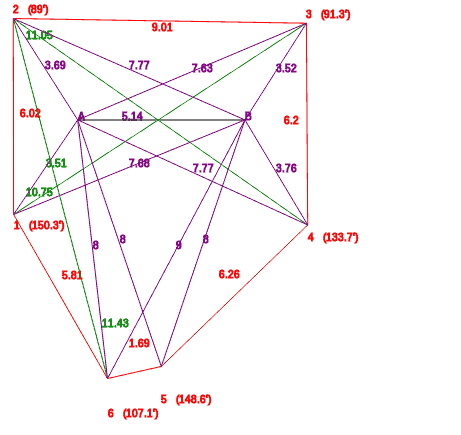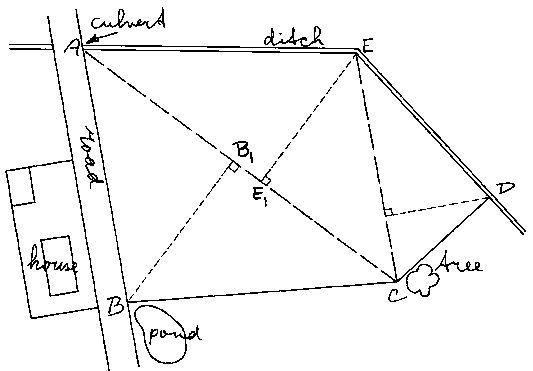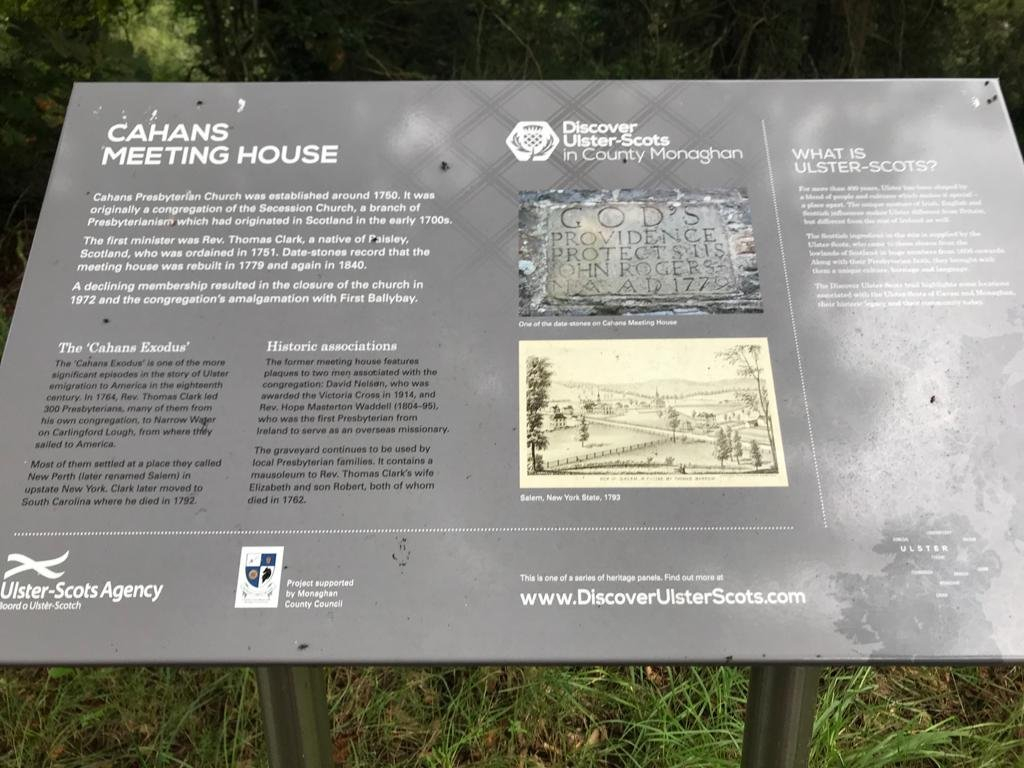Select Page

# Area Calculations

A common problem for a surveyor is the calculation of the surface area of a farmer’s field. The fields are often irregular which makes direct calculation of their areas difficult. In such case fields are divided into a number of regular areas (triangles, rectangles, etc.), of which the surfaces can be calculated with simple formulas. All areas are calculated separately and the sum of these areas gives the total area of the field.## Conservation Projects